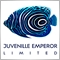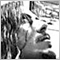# Draw Line in Future Time166

I'm stuck with this code, i have to find the highest and the lowest in like 161 candel, and that's ok, then i have to draw a vertical line  N candle forward where the price doesn't arrived yet , what is my error?

here is the code :

void M()

{

int max = iHighest(NULL,0,MODE_HIGH,161,0);

int min = iLowest(NULL,0,MODE_LOW,161,0);

datetime timemax = Time[max];

datetime timemin = Time[min];

ObjectCreate(NULL,"MAX",OBJ_VLINE,0,timemax,0);   // HOW CAN I SET THE FUTURE TIME/CANDLE ?

ObjectCreate(NULL,"MIN",OBJ_VLINE,0,timemin,0);

}Moderator
10313166

```void M()

{

int max = iHighest(NULL,0,MODE_HIGH,161,0);

int min = iLowest(NULL,0,MODE_LOW,161,0);

datetime timemax = Time[max];

datetime timemin = Time[min];

ObjectCreate(NULL,"MAX",OBJ_VLINE,0,timemax,0);   // HOW CAN I SET THE FUTURE TIME/CANDLE ?

ObjectCreate(NULL,"MIN",OBJ_VLINE,0,timemin,0);

}```20228

1. When you post code please use the CODE button (Alt-S)! (For large amounts of code, attach it.) Please edit your post.
General rules and best pratices of the Forum. - General - MQL5 programming forum
Messages Editor

Messages Editor

2. "How to set a future time?" Create a future time, e.g. Time + n * PeriodSeconds()37

Fede98:

I'm stuck with this code, i have to find the highest and the lowest in like 161 candel, and that's ok, then i have to draw a vertical line  N candle forward where the price doesn't arrived yet , what is my error?

here is the code :

void M()

{

int max = iHighest(NULL,0,MODE_HIGH,161,0);

int min = iLowest(NULL,0,MODE_LOW,161,0);

datetime timemax = Time[max];

datetime timemin = Time[min];

ObjectCreate(NULL,"MAX",OBJ_VLINE,0,timemax,0);   // HOW CAN I SET THE FUTURE TIME/CANDLE ?

ObjectCreate(NULL,"MIN",OBJ_VLINE,0,timemin,0);

}

```      //--------------------------------------------------------------------------------------------------------------||
// GetFutureTime
//--------------------------------------------------------------------------------------------------------------||

datetime CalcXYtoTime(int Xoffset)
{
datetime Xdate= 0;
int width=ChartGetInteger(0,CHART_WIDTH_IN_PIXELS,0);

int X =width-Xoffset ; int Y=0; int window= 0;
datetime xtim=0; double xpri=0;

ChartXYToTimePrice(0,X,Y,window,xtim,xpri);
Xdate= xtim;

return(Xdate);```

Assuming you alreay know the price.. thats the future time

with little change you can return the price as well :)))